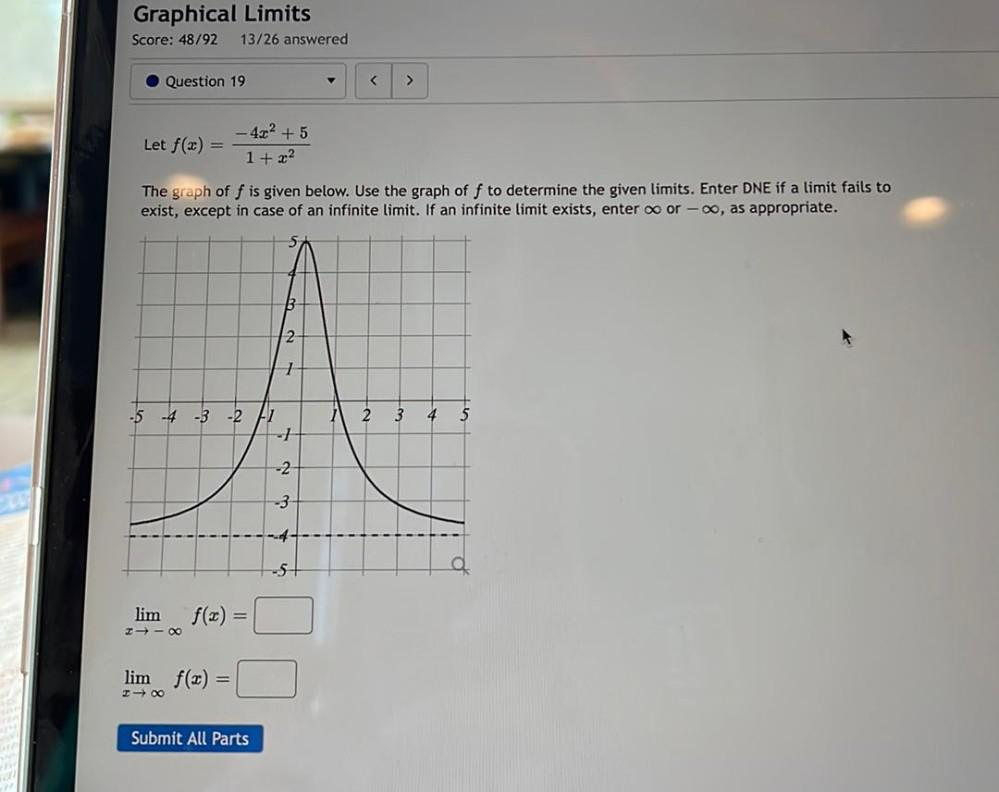Question:

# Let f(x) =– 4x² +5/1 +x² The graph of f is given below. Use the graph of f to determine the given limits. Enter DNE if a limit fails to exist, except in case of an infinite limit. If an infinite limitLet f(x) =– 4x² +5/1 +x² The graph of f is given below. Use the graph of f to determine the given limits. Enter DNE if a limit fails to exist, except in case of an infinite limit. If an infinite limit exists, enter ∞, or -∞, as appropriate. lim f(x)=________ x->-∞ lim f(x)=________ x->∞